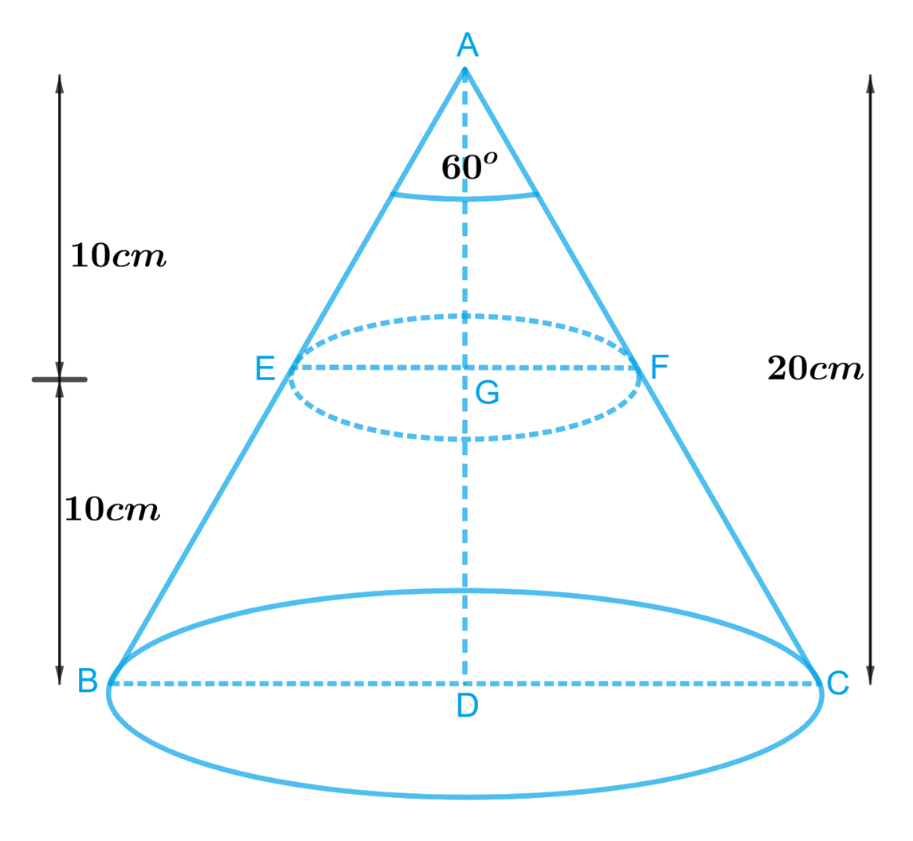# Ex.13.4 Q5 Surface Areas and Volumes Solution - NCERT Maths Class 10

Go back to  'Ex.13.4'

## Question

A metallic right circular cone $$20\,\rm{cm}$$ high and whose vertical angle is $$60^\circ$$ is cut into two parts at the middle of its height by a plane parallel to its base. If the frustum so obtained be drawn into a wire of diameter \begin{align}\frac{1}{{16}}\;\rm{cm}\end{align} Find the length of the wire.

Video Solution
Surface Areas And Volumes
Ex 13.4 | Question 5

## Text Solution

What is Known?

(i) A metallic right circular cone of height $$=20\;\rm{cm}$$ and whose vertical angle $$= 60^\circ$$ is cut into $$2$$ parts at the middle of its height by a plane parallel to its base

(ii) The frustum obtained is drawn into a wire of diameter \begin{align}=\frac{1}{{16}}\;\rm{cm}\end{align}

What is Unknown?

Length of the wire drawn out from a frustum

Reasoning:

Draw a figure to visualize the vertical cross section of a coneSince a metallic right circular cone is cut into two parts at the middle of its height by a plane parallel to its base.

From the figure it’s clear that

In , $$\Delta ABC$$ , $$EF$$ parallel to $$BC$$ and

\begin{align}AD &= 20cm\\AG &= 10cm\\\angle BAC &= 60^\circ \end{align}

To get the values of radii of both ends of the frustum formed compare $$\Delta ADB$$ and $$\Delta ADC$$

\begin{align}AD &= AD\,\left ( {{\text{Common}}} \right)\\AB &= AC\,\left( {{\text{Slant height}}} \right)\\\angle ADB &= \angle ADC = {90^{\rm{o}}}{\rm{ }}\,\, \left( {{\text{Right circular cone}}} \right)\\\Delta ADB &\cong \Delta ADC\, \left( {{\text{RHS criterion of congruency}}} \right)\\\angle BAD &= \angle DAC\,\left( {{\text{CPCT}}}\right)\end{align}

Then,

\begin{align}\angle BAD &= \angle DAC \\&= \frac{1}{2}\angle BAC \\&= \frac{1}{2} \times 60^\circ = 30^\circ \end{align}

In $$\Delta ADB$$

\begin{align}\frac{{BD}}{{AD}} &= \tan 30^\circ \\BD &= AD\tan 30^\circ \end{align}

Radius of lower end \begin{align}BD = \frac{{AD}}{{\sqrt 3 }}\end{align}

Similarly, in $$\Delta AEG$$

\begin{align}\frac{{EG}}{{AG}} &= \tan 30^\circ \\EG &= AG\tan 30^\circ \end{align}

Radius of upper end \begin{align}EG = \frac{{AG}}{{\sqrt 3 }}\end{align}

Now radii and height are known, finding volume of frustum of cone is easy

Since the frustum obtained is drawn into the wire which will be cylindrical in the shape.

Then the volume of the wire will be same as the volume of the frustum of the cone.

Therefore, Volume of the wire $$=$$ Volume of frustum of the cone

We will find the volume of the frustum by using formula;

Volume of frustum of a cone \begin{align} = \frac{1}{3}\pi h\left( {r_1^2 + r_2^2 + {r_1}{r_2}} \right)\end{align}

where $$r1, r2$$ and $$h$$ are the radii and height of the frustum of the cone respectively.

We will find the volume of the wire by using formula;

Volume of cylinder $$= \pi {r^2}h$$

where $$r$$ and $$h$$ are radius and height of the cylinder.

Steps:

In ,$$\Delta ABC$$ $$EF$$ parallel to $$BC$$ and

\begin{align}AD &= 20cm\\AG &= 10cm\\\angle BAC &= 60^\circ \end{align}

To get the values of radii of both ends of the frustum formed compare $$\Delta ADB$$ and $$\Delta ADC$$

\begin{align}AD &= AD\,\left ( {{\text{Common}}} \right)\\AB &= AC\,\left( {{\text{Slant height}}} \right)\\\angle ADB &= \angle ADC = {90^{\rm{o}}}{\rm{ }}\,\, \left( {{\text{Right circular cone}}} \right)\\\Delta ADB &\cong \Delta ADC\, \left( {{\text{RHS criterion of congruency}}} \right)\\\angle BAD &= \angle DAC\,\,\,\left( {{\text{CPCT}}}\right)\end{align}

Then,

\begin{align}\angle BAD &= \angle DAC \\&= \frac{1}{2}\angle BAC\\& = \frac{1}{2} \times 60^\circ = 30^\circ \end{align}

In $$\Delta ADB$$

\begin{align}\frac{{BD}}{{AD}} &= \tan 30^\circ \\BD &= AD\tan 30^\circ \\BD &= 20cm \times \frac{1}{{\sqrt 3 }}\\BD &= \frac{{20\sqrt 3 }}{3}cm\end{align}

Similarly, in $$\Delta AEG$$

\begin{align}\frac{{EG}}{{AG}} &= \tan 30^\circ \\EG &= AG\tan 30^\circ \\EG &= 10cm \times \frac{1}{{\sqrt 3 }}\\EG &= \frac{{10\sqrt 3 }}{3}cm\end{align}

Height of the frustum of the cone,$$h = 10cm$$

Radius of lower end,\begin{align}{r_1} = \frac{{20\sqrt 3 }}{3}cm\end{align}

Radius of upper end,\begin{align}{r_2} = \frac{{10\sqrt 3 }}{3}cm\end{align}

Diameter of the cylindrical wire,\begin{align}d = \frac{1}{{16}}cm\end{align}

Radius of the cylindrical wire,\begin{align}r = \frac{1}{2} \times \frac{1}{{16}}cm = \frac{1}{{32}}cm\end{align}

Let the length of the wire be $$H$$

Since the frustum is drawn into wire

Volume of the cylindrical wire $$=$$ Volume of frustum of the cone

\begin{align}\pi {r^2}H &= \frac{1}{3}\pi h\left( {r_1^2 + r_2^2 + {r_1}{r_2}} \right)\\H &= \frac{h}{{3{r^2}}}\left( {r_1^2 + r_2^2 + {r_1}{r_2}} \right)\\&= \frac{{10cm}}{{3 \times {{\left( {\frac{1}{{32}}cm} \right)}^2}}} \times \left[ {{{\left( {\frac{{20\sqrt 3 }}{3}cm} \right)}^2} + {{\left( {\frac{{10\sqrt 3 }}{3}cm} \right)}^2} + \frac{{20\sqrt 3 }}{3}cm \times \frac{{10\sqrt 3 }}{3}cm} \right]\\&= \frac{{10240}}{{3cm}} \times \left[ {\frac{{400}}{3}c{m^2} + \frac{{100}}{3}c{m^2} + \frac{{200}}{3}c{m^2}} \right]\\&= \frac{{10240}}{{3cm}} \times \frac{{700c{m^2}}}{3}\\&= \frac{{7168000}}{{9 \times 100}}m\\&= 7964.4m\end{align}

Length of the wire is $$7964.4m$$

Learn from the best math teachers and top your exams

• Live one on one classroom and doubt clearing
• Practice worksheets in and after class for conceptual clarity
• Personalized curriculum to keep up with school